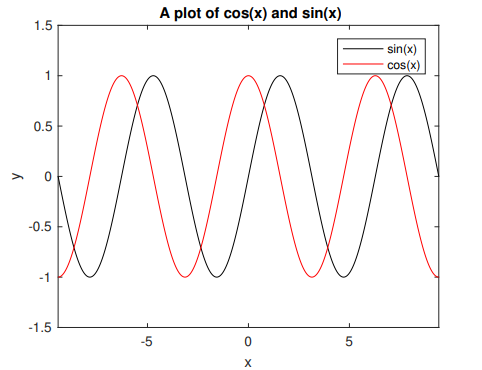# Matlab

CUNY offers a campus-wide license to MATLAB, Simulink, and all companion products. All faculty, researchers, and students are eligible to download and install these products on their university computers as well as their personally-owned computers.• You need to have a valid CUNY email address, either in the format citymail or ccny email.
• You need to renew license in your Matlab application once a year at the end of November.

### Preparation: Mathworks account

If you don’t have a Mathworks account associated with your CUNY email address yet, go to the following URL to create and account:

Levich InstituteJunjun Maojmao@ccny.cuny.edu212-650-6845
Chemical EngineeringAndrew Engaeng@ccny.cuny.edu212-650-6624## Installation

### Run the installer

When you run the matlab installer, choose installation method with “Log in with a MathWorks Account”.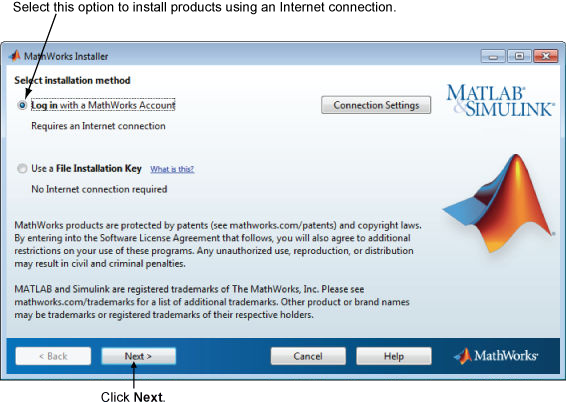1111017 Standard – Individual

The license renewal option is hidden under Help -> Licensing -> Update Current Licenses. You will need to do this every year at the end of November or earl December.

## Servers with preinstalled Matlab

Levich Institute and Chemical Engineering department offer following computing resources that have preinstalled Matlab. Matlab runs best on your own computer but the servers fit situation like:

• One needs to run prolonged sessions of Matlab jobs
• One’s Matlab jobs require memory and CPU more than a personal computer can handle.

### Cheme Linux server

Server Namecheme.engr.ccny.cuny.edu
Operating systemUbuntu 18.04
CPU64
RAM196 GB
Remote Accessssh, vmhub
Account ApplicationJunjun Mao <jmao@ccny.cuny.edu>

### Poincare Linux server

Server Namepoincare.engr.ccny.cuny.edu
Operating systemUbuntu 10.04
CPU32
RAM128 GB
Remote Accessssh, vmhub
Account ApplicationJunjun Mao <jmao@ccny.cuny.edu>

### Chemical Engineering Citrix server

Server Namecheportal.ccny.cuny.edu
Operating systemWindows
CPU2
RAM8 GB
Remote Accesshttps://cheportal.ccny.cuny.edu
Account ApplicationAndrew Eng <aeng@ccny.cuny.edu>
RemarkThis server offers Matlab through non-persistent virtual desktop

Note:

You need City College VPN account to access the server cheme.engr.ccny.cuny.edu and poincare.engr.ccny.cuny.edu by ssh.

## Matlab Online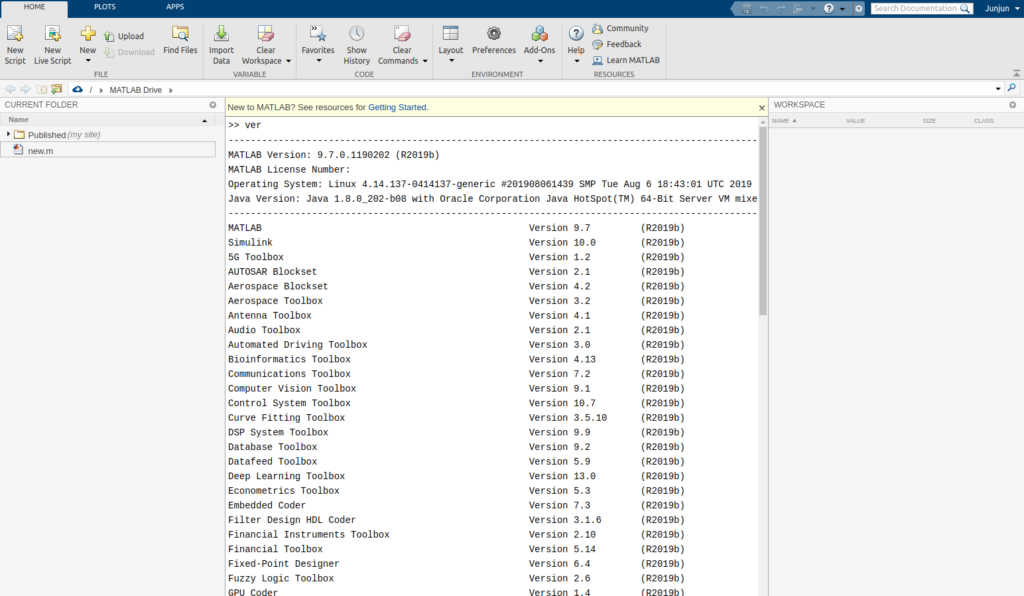You can run Matlab Online provided by Mathworks within a browser window if you have a Mathworks account with CCNY site license.

## Learning Matlab

### Online resources

• Matlab Onramp: Learn the essentials of MATLAB® through this free, two-hour introductory interactive tutorial on commonly used features and workflows.
• MIT Open course: Short videos serve as introduction to Matlab programming for beginners.

### Matlab books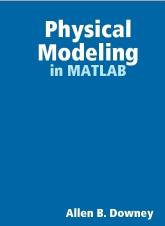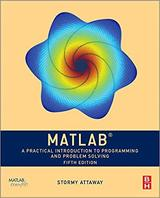## Matlab Quick Reference

### The Matlab Work Environment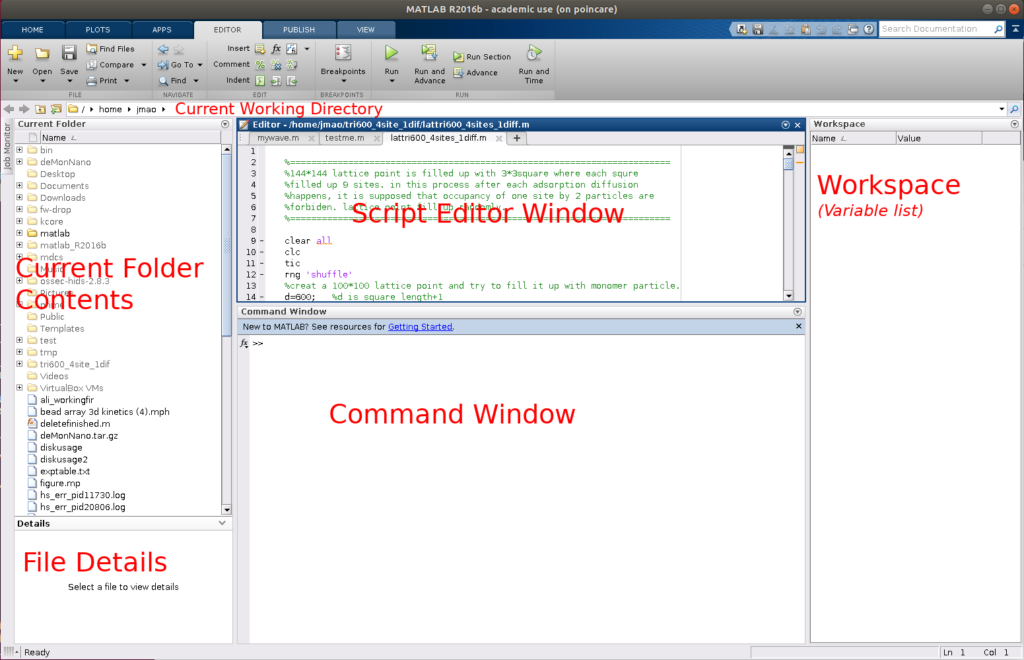### General Commands

Tip

Some commands have links to syntax details and examples.

CommandDescription
clcClear Command Window
clearRemove items from workspace, freeing up system memory
close allClose all plots
doc functionDisplay documentation page in Help browser
helpHelp for functions in Command Window
verVersion information for MathWorks products
% some comment textComment text
CTRL+CAbort the current operation
format shortDisplay 4 decimal places
format longDisplay 15 decimal places
disp(‘some text’)Print some text

### Defining Variables

CommandDescription
a = 3Define variable a to be 3
x = [1, 2, 3]Set x to be the row vector [1, 2, 3]
x = [1; 2; 3]Set x to be the column vector [1, 2, 3]T
A = [1, 2, 3, 4, 5, 6;
7, 8, 9, 10, 11, 12]
Set A to be a 2 × 6 matrix
x(2) = 7Change x from [1, 2, 3] to [1, 7, 3]
A(2,1) = 0Change A2,1 from 5 to 0

### Basic Arithmetic

CommandDescription
3*4, 7+4, 2-6, 8/3multiply, add, subtract and divide
3^7Compute 37
sqrt(5)Compute 5
log(3)Compute natural logarithm ln(3)
log10(100)Compute common logarithm (base 10) log(10)
abs(-5)Compute |-5|
sin(5*pi/3)Compute sin(5π/3)

### Round numbers

CommandDescription
floor(-1.5)-2; Round toward negative infinity
ceil(-1.5)-1; Round toward positive infinity
fix(-1.5)-1; Round toward zero
round(-1.5)-2; Round to nearest decimal or integer

### Matrices and Vectors

CommandDescription
zeros(12, 5)Make a 12 × 5 matrix of zeros
ones(12, 5)Make a 12 × 5 matrix of ones
eye(5)Make a 5 × 5 identity matrix
eye(12, 5)Make a 12 × 5 identity matrix
linspace(1.4, 6.3, 1004)Make a vector with 1004 elements evenly spaced between 1.4 and 6.3
logspace(1.4, 6.3, 1004)Make a vector with 1004 elements where the log of the spacing is
evenly increasing between 1.4 and 6.3
7:15Row vector of 7, 8, . . . , 14, 15

### Operations on Matrices and Vectors

CommandDescription
3 * xMultiply every element of x by 3
x + 2Add 2 to every element of x
x + yElement-wise addition of two vectors x and y
A * yProduct of a matrix and vector
A * BProduct of two matrices
A .* BElement-wise product of two matrices
A ^ 3Square matrix A to the third power
A .^ 3Every element of A to the third power
cos(A)Compute the cosine of every element of A
abs(A)Compute the absolute values of every element of A
A’Transpose of A
inv(A)Compute the inverse of A
det(A)Compute the determinant of A
eig(A)Compute the eigenvalues of A
size(A)Get the size of A

### Slicing Matrices and vectors

CommandDescription
x(2:12)The 2nd to the 12th elements of x
x(2:end)The 2nd to the last elements of x
x(1:3:end)Every third element of x from the first to last
A(5,:)Get the 5th row of A
A(:,5)Get the 5th column of A
A(5, 1:3)Get the first to third elements in the 5th row

### Constants

CommandDescription
piπ = 3.141592653589793
NaNNot a number (i.e. 0/0)
InfInfinity
realmaxLargest positive floating-point number 1.7977×10308
realminSmallest positive floating-point number 2.2251×10−308

### Loops

For loop:

for k = 1:5
disp(k);
end


While loop:

k = 0;
while k < 7
k = k + 1;
end


### Logicals

a = 10
a == 5 % Test if a is equal to 5
false
a == 10 % Test if a is equal to 10
true
a >= 5 % Test if a is greater than or equal to 5
true
a < 11 % Test if a is less than 11
true
a ~= 4 % Test if a is not equal to 4
true
a > 1 && a ~= 10 % Test if a is greater than 1 AND not equal to 10
false
a > 1 || a ~= 10 % Test if a is greater than 1 OR not equal to 10
true


### Conditional Statements

if a > 10
disp('Greater than 10');
elseif a == 5
disp('a is 5');
else
disp('Neither condition met');
end


### Functions

function output = addNumbers(x, y)
output = x + y;
end

5


### Plotting

CommandDescription
plot(x,y)Plot y versus x (must be the same length)
loglog(x,y)Plot y versus x on a log-log scale (both axes have a logarithmic scale)
semilogx(x, y)Plot y versus x with x on a log scale
semilogy(x, y)Plot y versus x with y on a log scale
axis equalForce the x and y axes to be scaled equally
title(‘A Title’)Add a title to the plot
xlabel(‘x label’)Add a label to the x axis
ylabel(‘y label’)Add a label to the y axis
legend(‘foo’, ‘bar’)Label 2 curves for the plot
gridAdd a grid to the plot
hold onMultiple plots on single figure
figureStart a new plot

Example:

x = linspace(-3*pi, 3*pi, 1000);
y1 = sin(x);
y2 = cos(x);

plot(x, y1, 'k-');  % Plot sin(x) as a black line
hold on             % Now we can add another curve
plot(x, y2, 'r-');  % Plot cos(x) as a red line

% Set the axis limits
axis([-3*pi, 3*pi, -1.5, 1.5])

legend('sin(x)', 'cos(x)');